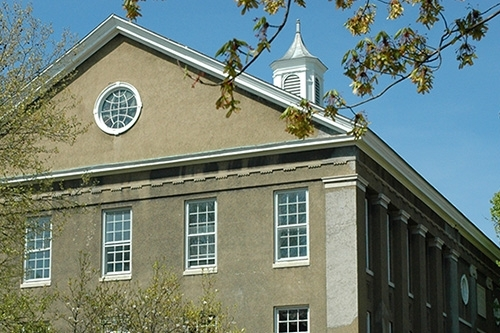Mathematics Department

# Junqing Qian

Job Title
Assistant Professor of Mathematics
Bailey Hall 108D

## Research interests

My research interest is at the intersection of number theory and differential geometry. More precisely, I am primarily interested in capturing algebraic information to help with problems in geometry and geometric analysis. For example, I discovered a connection between the Kähler–Einstein metric on punctured spheres, a subject in differential geometry, and modular functions, a subject in number theory; this connection overcame the obstacle from other methods and settled the metric problem, a problem in geometry. World-leading mathematicians projected the existence and importance of further connections between the two different fields. Some discovered ones have been applied in physics, such as string theory. Naturally, I am also interested in topics in both fields. On the algebraic side, I am interested in studying and developing the theories of arithmetic differential equations and geometry; on the analytic side, I am interested in Calabi flow on toric manifolds and exploring mathematical physics.